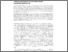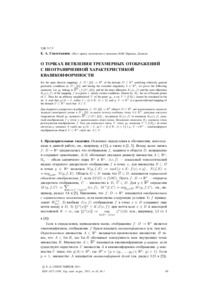# О точках ветвления трехмерных отображений с неограниченной характеристикой квазикомфорности

Севостьянов, Е. А. (2011) О точках ветвления трехмерных отображений с неограниченной характеристикой квазикомфорности. Український математичний журнал, 63 (1). pp. 69-79. ISSN 1027-3190Preview
Text

## Abstract

For open discrete mappings f WD n fbg ! R3 of a domain D � R3 satisfying relatively general geometric conditions in D n fbg and having an essential singularity at a point b 2 R3; we prove the following statement: Let a point y0 belong to R3 n f .D n fbg/ and let the inner dilatation KI .x; f / and outer dilatation KO.x; f / of the mapping f at the point x satisfy certain conditions. Let Bf denote the set of branch points of the mapping f: Then, for an arbitrary neighborhood V of the point y0; the set V \f .Bf / cannot be contained in a set A such that g.A/ D I; where I D ft 2 RW jt j < 1g and gWU ! Rn is a quasiconformal mapping of a domain U � Rn such that A � U:

Item Type: Article Q Science > QA Mathematics > Mathematical Analysis Faculty of Physics and Mathematics > Department of Mathematical Analysis Ірина Ігорівна Таргонська 10 Nov 2014 09:14 01 Mar 2017 08:50 http://eprints.zu.edu.ua/id/eprint/13839View Item